$$\newcommand{\dt}{\Delta t} \newcommand{\tp}{\thinspace .} \newcommand{\uex}{{u_{\small\mbox{e}}}} \newcommand{\x}{\boldsymbol{x}} \renewcommand{\u}{\boldsymbol{u}} \newcommand{\dx}{\, \mathrm{d}x} \newcommand{\ds}{\, \mathrm{d}s} \newcommand{\Real}{\mathbb{R}} \newcommand{\ub}{u_{_\mathrm{D}}} \newcommand{\GD}{\Gamma_{_\mathrm{D}}} \newcommand{\GN}{\Gamma_{_\mathrm{N}}} \newcommand{\GR}{\Gamma_{_\mathrm{R}}} \newcommand{\inner}{\langle #1, #2 \rangle} \newcommand{\renni}{\langle #2, #1 \rangle}$$

# FEniCS implementation

## The complete program

A FEniCS program for solving our test problem for the Poisson equation in 2D with the given choices of $$\ub$$, $$f$$, and $$\Omega$$ may look as follows:

from fenics import *

# Create mesh and define function space
mesh = UnitSquareMesh(8, 8)
V = FunctionSpace(mesh, 'P', 1)

# Define boundary condition
u_D = Expression('1 + x*x + 2*x*x', degree=2)

def boundary(x, on_boundary):
return on_boundary

bc = DirichletBC(V, u_D, boundary)

# Define variational problem
u = TrialFunction(V)
v = TestFunction(V)
f = Constant(-6.0)
L = f*v*dx

# Compute solution
u = Function(V)
solve(a == L, u, bc)

# Plot solution
u.rename('u', 'solution')
plot(u)
plot(mesh)

# Save solution to file in VTK format
vtkfile = File('poisson.pvd')
vtkfile << u

# Compute error in L2 norm
error_L2 = errornorm(u_D, u, 'L2')

# Compute maximum error at vertices
vertex_values_u_D = u_D.compute_vertex_values(mesh)
vertex_values_u = u.compute_vertex_values(mesh)
import numpy as np
error_max = np.max(np.abs(vertex_values_u_D - vertex_values_u))

# Print errors
print('error_L2  =', error_L2)
print('error_max =', error_max)

# Hold plot
interactive()


The complete code can be found in the file ft01_poisson.py.

## Running the program

The FEniCS program must be available in a plain text file, written with a text editor such as Atom, Sublime Text, Emacs, Vim, or similar.

There are several ways to run a Python program like ft01_poisson.py:

• Use a terminal window.
• Use an integrated development environment (IDE), e.g., Spyder.
• Use a Jupyter notebook.

### Terminal window

Open a terminal window, move to the directory containing the program and type the following command:

Terminal> python ft01_poisson.py


Note that this command must be run in a FEniCS-enabled terminal. For users of the FEniCS Docker containers, this means that you must type this command after you have started a FEniCS session using fenicsproject run or fenicsproject start.

When running the above command, FEniCS will run the program to compute the approximate solution $$u$$. The approximate solution $$u$$ will be compared to the exact solution $$\uex$$ and the error in the $$L^2$$ and maximum norms will be printed. Since we know that our approximate solution should reproduce the exact solution to within machine precision, this error should be small, something on the order of $$10^{-15}$$.

Figure 1: Plot of the solution in the first FEniCS example.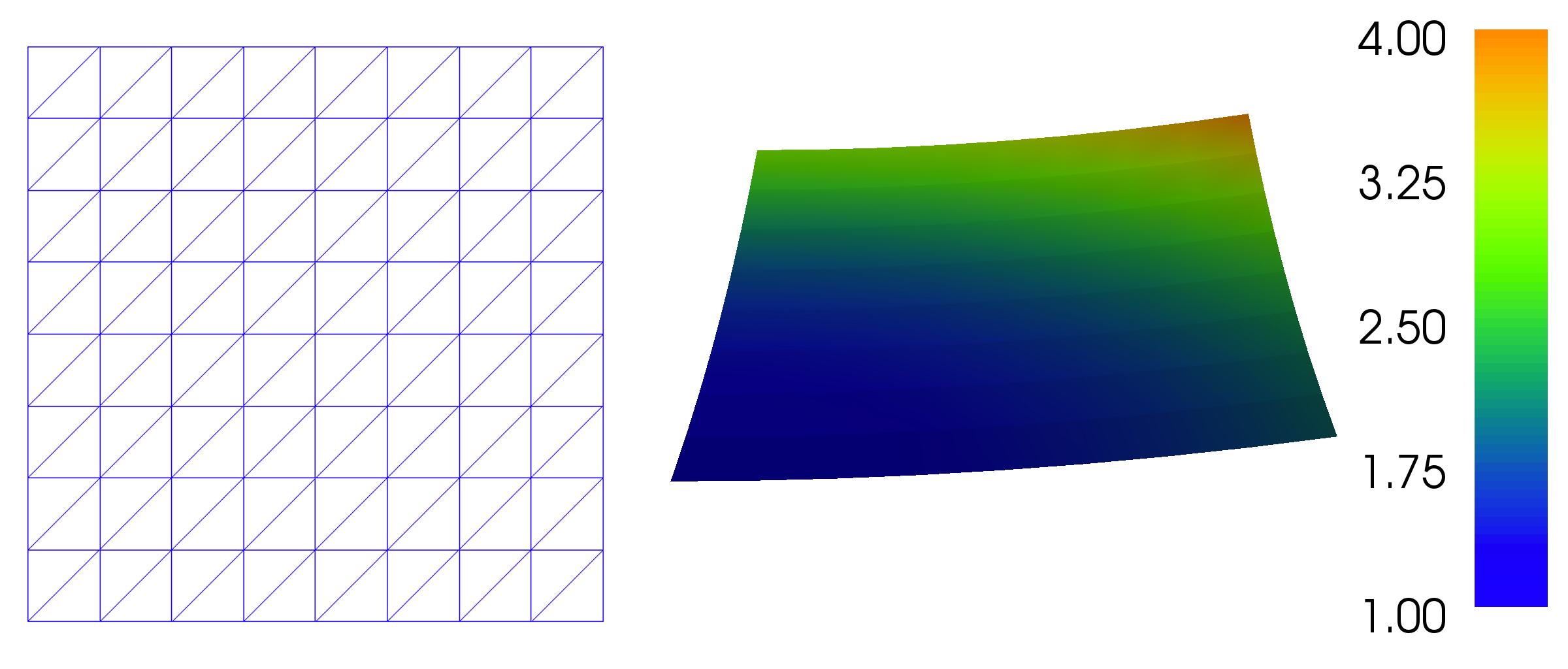### Spyder

Many prefer to work in an integrated development environment that provides an editor for programming, a window for executing code, a window for inspecting objects, etc. The Spyder tool comes with all major Python installations. Just open the file ft01_poisson.py and press the play button to run it. We refer to the Spyder tutorial to learn more about working in the Spyder environment. Spyder is highly recommended if you are used to working in the graphical MATLAB environment.

### Jupyter notebooks

Notebooks make it possible to mix text and executable code in the same document, but you can also just use it to run programs in a web browser. Start jupyter notebook from a terminal window, find the New pulldown menu in the upper right part of the GUI, choose a new notebook in Python 2 or 3, write %load ft01_poisson.py in the blank cell of this notebook, then press Shift+Enter to execute the cell. The file ft01_poisson.py will then be loaded into the notebook. Re-execute the cell (Shift+Enter) to run the program. You may divide the entire program into several cells to examine intermediate results: place the cursor where you want to split the cell and choose Edit - Split Cell.

# Dissection of the program

We shall now dissect our FEniCS program in detail. The listed FEniCS program defines a finite element mesh, a finite element function space $$V$$ on this mesh, boundary conditions for $$u$$ (the function $$\ub$$), and the bilinear and linear forms $$a(u,v)$$ and $$L(v)$$. Thereafter, the unknown trial function $$u$$ is computed. Then we can compare the numerical and exact solution as well as visualize the computed solution $$u$$.

## The important first line

The first line in the program,

from fenics import *


imports the key classes UnitSquareMesh, FunctionSpace, Function, and so forth, from the FEniCS library. All FEniCS programs for solving PDEs by the finite element method normally start with this line.

## Generating simple meshes

The statement

mesh = UnitSquareMesh(8, 8)


defines a uniform finite element mesh over the unit square $$[0,1]\times [0,1]$$. The mesh consists of cells, which in 2D are triangles with straight sides. The parameters 8 and 8 specify that the square should be divided into $$8\times 8$$ rectangles, each divided into a pair of triangles. The total number of triangles (cells) thus becomes 128. The total number of vertices in the mesh is $$9\cdot 9=81$$. In later chapters, you will learn how to generate more complex meshes.

## Defining the finite element function space

Having a mesh, we can define a finite element function space V over this mesh:

V = FunctionSpace(mesh, 'P', 1)


The second argument 'P' specifies the type of element, while the third argument is the degree of the basis functions of the element. The type of element is here $$\mathsf{P}$$, implying the standard Lagrange family of elements. You may also use 'Lagrange' to specify this type of element. FEniCS supports all simplex element families and the notation defined in the Periodic Table of the Finite Elements .

The third argument 1 specifies the degree of the finite element. In this case, the standard $$\mathsf{P}_1$$ linear Lagrange element, which is a triangle with nodes at the three vertices. Some finite element practitioners refer to this element as the "linear triangle". The computed solution $$u$$ will be continuous and linearly varying in $$x$$ and $$y$$ over each cell in the mesh. Higher-degree polynomial approximations over each cell are trivially obtained by increasing the third parameter to FunctionSpace, which will then generate function spaces of type $$\mathsf{P}_2$$, $$\mathsf{P}_3$$, and so forth. Changing the second parameter to 'DP' creates a function space for discontinuous Galerkin methods.

## Defining the trial and test functions

In mathematics, we distinguish between the trial and test spaces $$V$$ and $$\hat{V}$$. The only difference in the present problem is the boundary conditions. In FEniCS we do not specify the boundary conditions as part of the function space, so it is sufficient to work with one common space V for the trial and test functions in the program:

u = TrialFunction(V)
v = TestFunction(V)


## Defining the boundary and the boundary conditions

The next step is to specify the boundary condition: $$u=\ub$$ on $$\partial\Omega$$. This is done by

bc = DirichletBC(V, u_D, boundary)


where u_D is an expression defining the solution values on the boundary, and boundary is a function (or object) defining which points belong to the boundary.

Boundary conditions of the type $$u=\ub$$ are known as Dirichlet conditions. For the present finite element method for the Poisson problem, they are also called essential boundary conditions, as they need to be imposed explicitly as part of the trial space (in contrast to being defined implicitly as part of the variational formulation). Naturally, the FEniCS class used to define Dirichlet boundary conditions is named DirichletBC.

The variable u_D refers to an Expression object, which is used to represent a mathematical function. The typical construction is

u_D = Expression(formula, degree=1)


where formula is a string containing the mathematical expression. This formula is written with C++ syntax. The expression is automatically turned into an efficient, compiled C++ function. The second argument degree is a parameter that specifies how the expression should be treated in computations. FEniCS will interpolate the expression into some finite element space. It is usually a good choice to interpolate expressions into the same space $$V$$ that is used for the trial and test functions, but in certain cases, one may want to use a more accurate (higher degree) representation of expressions.

The expression may depend on the variables x and x corresponding to the $$x$$ and $$y$$ coordinates. In 3D, the expression may also depend on the variable x corresponding to the $$z$$ coordinate. With our choice of $$\ub(x,y)=1 + x^2 + 2y^2$$, the formula string can be written as 1 + x*x + 2*x*x:

u_D = Expression('1 + x*x + 2*x*x', degree=2)


We set the degree to $$2$$ so that u_D may represent the exact quadratic solution to our test problem.

(hpl 1: What do you do with a really smooth function line $$sin(x)$$? What degree? Why not a default degree? This is difficult for a beginner.)

String expressions must have valid C++ syntax! The string argument to an Expression object must obey C++ syntax. Most Python syntax for mathematical expressions is also valid C++ syntax, but power expressions make an exception: p**a must be written as pow(p, a) in C++ (this is also an alternative Python syntax). The following mathematical functions can be used directly in C++ expressions when defining Expression objects: cos, sin, tan, acos, asin, atan, atan2, cosh, sinh, tanh, exp, frexp, ldexp, log, log10, modf, pow, sqrt, ceil, fabs, floor, and fmod. Moreover, the number $$\pi$$ is available as the symbol pi. All the listed functions are taken from the cmath C++ header file, and one may hence consult the documentation of cmath for more information on the various functions.

If/else tests are possible using the C syntax for inline branching. The function $$f(x,y) = \left\lbrace\begin{array}{ll} x^2, & x, y\geq 0\\ 2, & \hbox{otherwise}\end{array}\right.$$ is implemented as

f = Expression('x >= 0 && x >= 0 ? pow(x, 2) : 2', degree=1)


Parameters in expression strings are allowed, but must be initialized via keyword arguments when creating the Expression object. For example, the function $$f(x)=e^{-\kappa\pi^2t}\sin(\pi k x)$$ can be coded as

f = Expression('exp(-kappa*pow(pi, 2)*t)*sin(pi*k*x)', degree=1,
kappa=1.0, t=0, k=4)


At any time, parameters can be updated:

f.t += dt
f.k = 10


The function boundary specifies which points that belong to the part of the boundary where the boundary condition should be applied:

def boundary(x, on_boundary):
return on_boundary


A function like boundary for marking the boundary must return a boolean value: True if the given point x lies on the Dirichlet boundary and False otherwise. The argument on_boundary is True if x is on the physical boundary of the mesh, so in the present case, where we are supposed to return True for all points on the boundary, we can just return the supplied value of on_boundary. The boundary function will be called for every discrete point in the mesh, which allows us to have boundaries where $$u$$ are known also inside the domain, if desired.

One way to think about the specification of boundaries in FEniCS is that FEniCS will ask you (or rather the function boundary which you have implemented) whether or not a specific point x is part of the boundary. FEniCS already knows whether the point belongs to the actual boundary (the mathematical boundary of the domain) and kindly shares this information with you in the variable on_boundary. You may choose to use this information (as we do here), or ignore it completely.

The argument on_boundary may also be omitted, but in that case we need to test on the value of the coordinates in x:

def boundary(x):
return x == 0 or x == 0 or x == 1 or x == 1


Comparing floating-point values using an exact match test with == is not good programming practice, because small round-off errors in the computations of the x values could make a test x == 1 become false even though x lies on the boundary. A better test is to check for equality with a tolerance, either explicitly

def boundary(x):
return abs(x) < tol or abs(x) < tol \
or abs((x - 1) < tol or abs(x - 1) < tol


or with the near command in FEniCS:

def boundary(x):
return near(x, 0, tol) or near(x, 0, tol) \
or near(x, 1, tol) or near(x, 1, tol)


Never use == for comparing real numbers! A comparison like x == 1 should never be used if x is a real number, because rounding errors in x may make the test fail even when it is mathematically correct. Consider

>>> 0.1 + 0.2 == 0.3
False
>>> 0.1 + 0.2
0.30000000000000004


Comparison of real numbers needs to be made with tolerances! The values of the tolerances depend on the size of the numbers involved in arithmetic operations:

>>> abs(0.1 + 0.2 - 0.3)
5.551115123125783e-17
>>> abs(1.1 + 1.2 - 2.3)
0.0
>>> abs(10.1 + 10.2 - 20.3)
3.552713678800501e-15
>>> abs(100.1 + 100.2 - 200.3)
0.0
>>> abs(1000.1 + 1000.2 - 2000.3)
2.2737367544323206e-13
>>> abs(10000.1 + 10000.2 - 20000.3)
3.637978807091713e-12


For numbers of unit size, tolerances as low as $$3\cdot 10^{-16}$$ can be used (in fact, this tolerance is known as the constant DOLFIN_EPS in FEniCS). Otherwise, an appropriately scaled tolerance must be used.

## Defining the source term

Before defining the bilinear and linear forms $$a(u,v)$$ and $$L(v)$$ we have to specify the source term $$f$$:

f = Expression('-6', degree=1)


When $$f$$ is constant over the domain, f can be more efficiently represented as a Constant:

f = Constant(-6)


## Defining the variational problem

We now have all the ingredients we need to define the variational problem:

a = dot(grad(u), grad(v))*dx
L = f*v*dx


In essence, these two lines specify the PDE to be solved. Note the very close correspondence between the Python syntax and the mathematical formulas $$\nabla u\cdot\nabla v \dx$$ and $$fv \dx$$. This is a key strength of FEniCS: the formulas in the variational formulation translate directly to very similar Python code, a feature that makes it easy to specify and solve complicated PDE problems. The language used to express weak forms is called UFL (Unified Form Language)   and is an integral part of FEniCS.

## Forming and solving the linear system

Having defined the finite element variational problem and boundary condition, we can now ask FEniCS to compute the solution:

u = Function(V)
solve(a == L, u, bc)


Note that we first defined the variable u as a TrialFunction and used it to represent the unknown in the form a. Thereafter, we redefined u to be a Function object representing the solution; i.e., the computed finite element function $$u$$. This redefinition of the variable u is possible in Python and is often used in FEniCS applications for linear problems. The two types of objects that u refers to are equal from a mathematical point of view, and hence it is natural to use the same variable name for both objects.

## Plotting the solution

Once the solution has been computed, it can be visualized by the plot() command:

plot(u)
plot(mesh)
interactive()


Clicking on Help or typing h in the plot windows brings up a list of commands. For example, typing m brings up the mesh. With the left, middle, and right mouse buttons you can rotate, translate, and zoom (respectively) the plotted surface to better examine what the solution looks like. You must click Ctrl+q to kill the plot window and continue execution beyond the command interactive(). In the example program, we have therefore placed the call to interactive() at the very end. Alternatively, one may use the command plot(u, interactive=True) which again means you can interact with the plot window and that execution will be halted until the plot window is closed.

Figure 1 displays the resulting $$u$$ function.

## Exporting and post-processing the solution

It is also possible to save the computed solution to file for post-processing, e.g., in VTK format:

vtkfile = File('poisson.pvd')
vtkfile << u


The poisson.pvd file can now be loaded into any front-end to VTK, in particular ParaView or VisIt. The plot() function is intended for quick examination of the solution during program development. More in-depth visual investigations of finite element solutions will normally benefit from using highly professional tools such as ParaView and VisIt.

Prior to plotting and storing solutions to file it is wise to give u a proper name by u.rename('u', 'solution'). Then u will be used as name in plots (rather than the more cryptic default names like f_7).

Once the solution has been stored to file, it can be opened in Paraview by choosing File - Open. Find the file poisson.pvd, and click the green Apply button to the left in the GUI. A 2D color plot of $$u(x,y)$$ is then shown. You can save the figure to file by File - Export Scene... and choosing a suitable filename. For more information about how to install and use Paraview, see the Paraview web page.

Figure 2: Visualization of the solution of the test problem in ParaView, with contour lines added in the right plot.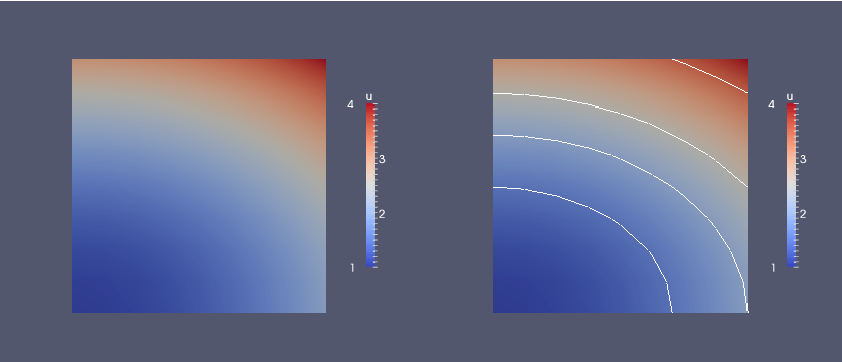## Computing the error

Finally, we compute the error to check the accuracy of the solution. We do this by comparing the finite element solution u with the exact solution u_D, which in this example happens to be the same as the Expression used to set the boundary conditions. We compute the error in two different ways. First, we compute the $$L^2$$ norm of the error, defined by $$E = \sqrt{\int_\Omega (\ub - u)^2\dx}\tp$$ Since the exact solution is quadratic and the finite element solution is piecewise linear, this error will be nonzero. To compute this error in FEniCS, we simply write

error_L2 = errornorm(u_D, u, 'L2')


The errornorm() function can also compute other error norms such as the $$H^1$$ norm. Type pydoc fenics.errornorm in a terminal window for details.

We also compute the maximum value of the error at all the vertices of the finite element mesh. As mentioned above, we expect this error to be zero to within machine precision for this particular example. To compute the error at the vertices, we first ask FEniCS to compute the value of both u_D and u at all vertices, and then subtract the results:

vertex_values_u_D = u_D.compute_vertex_values(mesh)
vertex_values_u = u.compute_vertex_values(mesh)
import numpy as np
error_max = np.max(np.abs(vertex_values_u_D - vertex_values_u))


We have here used the maximum and absolute value functions from numpy, because these are much more efficient for large arrays (a factor of 30) than Python's built-n max and abs functions.

How to check that the error vanishes. With inexact arithmetics, as we always have on a computer, the maximum error at the vertices is not zero, but should be a small number. The machine precision is about $$10^{-16}$$, but in finite element calculations, rounding errors of this size may accumulate, to produce an error larger than $$10^{-16}$$. Experiments show that increasing the number of elements and increasing the degree of the finite element polynomials increases the error. For a mesh with $$2\times(20\times 20)$$ cubic Lagrange elements (degree 3) the error is about $$2\cdot 10^{-12}$$, while for 81 linear elements the error is about $$2\cdot 10^{-15}$$.

## Examining degrees of freedom and vertex values

A finite element function like $$u$$ is expressed as a linear combination of basis functions $$\phi_j$$, spanning the space $$V$$: $$\begin{equation} u = \sum_{j=1}^N U_j \phi_j \tag{2.13}\tp \end{equation}$$ By writing solve(a == L, u, bc) in the program, a linear system will be formed from $$a$$ and $$L$$, and this system is solved for the values $$U_1,\ldots,U_N$$. The values $$U_1,\ldots,U_N$$ are known as the degrees of freedom ("dofs") or nodal values of $$u$$. For Lagrange elements (and many other element types) $$U_j$$ is simply the value of $$u$$ at the node with global number $$j$$. The locations of the nodes and cell vertices coincide for linear Lagrange elements, while for higher-order elements there are additional nodes associated with the facets, edges and sometimes also the interior of cells.

Having u represented as a Function object, we can either evaluate u(x) at any point x in the mesh (expensive operation!), or we can grab all the degrees of freedom in the vector $$U$$ directly by

nodal_values_u = u.vector()


The result is a Vector object, which is basically an encapsulation of the vector object used in the linear algebra package that is used to solve the linear system arising from the variational problem. Since we program in Python it is convenient to convert the Vector object to a standard numpy array for further processing:

array_u = nodal_values_u.array()


With numpy arrays we can write MATLAB-like code to analyze the data. Indexing is done with square brackets: array_u[j], where the index j always starts at 0. If the solution is computed with piecewise linear Lagrange elements ($$\mathsf{P}_1$$), then the size of the array array_u is equal to the number of vertices, and each array_u[j] is the value at some vertex in the mesh. However, the degrees of freedom are not necessarily numbered in the same way as the vertices of the mesh, see the section Examining the degrees of freedom for details. If we therefore want to know the values at the vertices, we need to call the function u.compute_vertex_values(). This function returns the values at all the vertices of the mesh as a numpy array with the same numbering as for the vertices of the mesh, for example:

vertex_values_u = u.compute_vertex_values()


Note that for $$\mathsf{P}_1$$ elements the arrays array_u and vertex_values_u have the same lengths and contain the same values, albeit in different order.

# Deflection of a membrane

Our first FEniCS program for the Poisson equation targeted a simple test problem where we could easily verify the implementation. Now we turn the attention to a more physically relevant problem, in a non-trivial geometry, and that results in solutions of somewhat more exciting shape.

We want to compute the deflection $$D(x,y)$$ of a two-dimensional, circular membrane, subject to a load $$p$$ over the membrane. The appropriate PDE model is $$\begin{equation} -T\nabla^2 D = p(x,y)\quad\hbox{in }\Omega = \{ (x,y)\,\vert\, x^2+y^2\leq R\}\tp \tag{2.14} \end{equation}$$ Here, $$T$$ is the tension in the membrane (constant), and $$p$$ is the external pressure load. The boundary of the membrane has no deflection, implying $$D=0$$ as boundary condition. A localized load can be modeled as a Gaussian function: $$\begin{equation} p(x,y) = {A\over 2\pi\sigma}\exp{\left( - {1\over2}\left( {x-x_0\over\sigma}\right)^2 - {1\over2}\left( {y-y_0\over\sigma}\right)^2 \right)}\, . \tag{2.15} \end{equation}$$ The parameter $$A$$ is the amplitude of the pressure, $$(x_0,y_0)$$ the localization of the maximum point of the load, and $$\sigma$$ the "width" of $$p$$.

## Scaling the equation

The localization of the pressure, $$(x_0,y_0)$$, is for simplicity set to $$(0, R_0)$$. There are many physical parameters in this problem, and we can benefit from grouping them by means of scaling. Let us introduce dimensionless coordinates $$\bar x = x/R$$, $$\bar y = y/R$$, and a dimensionless deflection $$w=D/D_c$$, where $$D_c$$ is a characteristic size of the deflection. Introducing $$\bar R_0=R_0/R$$, we get $$-\frac{\partial^2 w}{\partial\bar x^2} -\frac{\partial^2 w}{\partial\bar y^2}= \alpha \exp{\left( - \beta^2(\bar x^2 + (\bar y-\bar R_0)^2)\right)}\hbox{ for } \bar x^2 + \bar y^2 < 1,$$ where $$\alpha = \frac{R^2A}{2\pi T D_c\sigma},\quad\beta = \frac{R}{\sqrt{2}\sigma}\tp$$ With an appropriate scaling, $$w$$ and its derivatives are of size unity, so the left-hand side of the scaled PDE is about unity in size, while the right-hand side has $$\alpha$$ as its characteristic size. This suggest choosing $$\alpha$$ to be unity, or around unit. We shall in this particular case choose $$\alpha=4$$. With this value, the solution is $$w(\bar x,\bar y) = 1-\bar x^2 - \bar y^2$$. (One can also find the analytical solution in scaled coordinates and show that the maximum deflection $$D(0,0)$$ is $$D_c$$ if we choose $$\alpha=4$$ to determine $$D_c$$.) With $$D_c=AR^2/(8\pi\sigma T)$$ and dropping the bars we get the scaled problem $$\begin{equation} -\nabla^2w = 4\exp{\left( - \beta^2(x^2 + (y-R_0)^2)\right)}, \tag{2.16} \end{equation}$$ to be solved over the unit circle with $$w=0$$ on the boundary. Now there are only two parameters to vary: the dimensionless extent of the pressure, $$\beta$$, and the localization of the pressure peak, $$R_0\in [0,1]$$. As $$\beta\rightarrow 0$$, we have a special case with solution $$w=1-x^2-y^2$$.

Given a computed scaled solution $$w$$, the physical deflection can be computed by $$D = \frac{AR^2}{8\pi\sigma T}w\tp$$

Just a few modifications are necessary in our previous program to solve this new problem.

## Defining the mesh

A mesh over the unit circle can be created by the mshr tool in FEniCS:

from mshr import *
domain = Circle(Point(0.0, 0.0), 1.0)
n = 20
mesh = generate_mesh(domain, n)
plot(mesh, interactive=True)


The Circle shape from mshr takes the center and radius of the circle as the two first arguments, while n is the resolution, here the suggested number of cells per radius.

The right-hand side pressure function is represented by an Expression object. There are two physical parameters in the formula for $$f$$ that enter the expression string and these parameters must have their values set by keyword arguments:

beta = 8
R0 = 0.6
p = Expression(
'4*exp(-pow(beta,2)*(pow(x, 2) + pow(x-R0, 2)))',
beta=beta, R0=R0)


The coordinates in Expression objects must be a vector with indices 0, 1, and 2, and with the name x. Otherwise we are free to introduce names of parameters as long as these are given default values by keyword arguments. All the parameters initialized by keyword arguments can at any time have their values modified. For example, we may set

p.beta = 12
p.R0 = 0.3


## Defining the variational problem

We may introduce w instead of u as primary unknown and p instead of f as right-hand side function:

w = TrialFunction(V)
v = TestFunction(V)
L = p*v*dx

w = Function(V)
solve(a == L, w, bc)


## Plotting the solution

It is of interest to visualize the pressure $$p$$ along with the deflection $$w$$ so that we can examine membrane's response to the pressure. We must then transform the formula (Expression) to a finite element function (Function). The most natural approach is to construct a finite element function whose degrees of freedom are calculated from $$p$$. That is, we interpolate $$p$$:

p = interpolate(p, V)


Note that the assignment to p destroys the previous Expression object p, so if it is of interest to still have access to this object, another name must be used for the Function object returned by interpolate.

We can now plot w and p on the screen as well as save the fields to file in VTK format:

plot(w, title='Deflection')

vtkfile_w = File('membrane_deflection.pvd')
vtkfile_w << w
vtkfile_p << p


Figure 3 shows the result of the plot commands.

Figure 3: Load (left) and resulting deflection (right) of a circular membrane.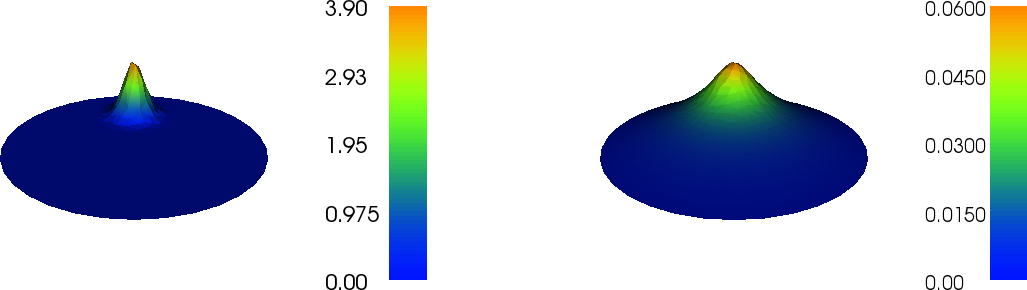## Making curve plots through the domain

The best way to compare the load and the deflection is to make a curve plot along the line $$x=0$$. This is just a matter of defining a set of points along the line and evaluating the finite element functions w and p at these points:

# Curve plot along x = 0 comparing p and w
import numpy as np
import matplotlib.pyplot as plt
tol = 1E-8  # avoid hitting points outside the domain
y = np.linspace(-1+tol, 1-tol, 101)
points = [(0, y_) for y_ in y]  # 2D points
w_line = np.array([w(point) for point in points])
p_line = np.array([p(point) for point in points])
plt.plot(y, 100*w_line, 'r-', y, p_line, 'b--') # magnify w
plt.legend(['100 x deflection', 'load'], loc='upper left')
plt.xlabel('y'); plt.ylabel('$p$ and $100u$')
plt.savefig('plot.pdf'); plt.savefig('plot.png')

# Hold plots
interactive()
plt.show()


The complete code can be found in the file ft02_poisson_membrane.py.

The resulting curve plot appears in Figure 4. It is seen how the localized input ($$p$$) is heavily damped and smoothened in the output ($$w$$). This reflects a typical property of the Poisson equation.

Figure 4: Comparison of membrane load and deflection.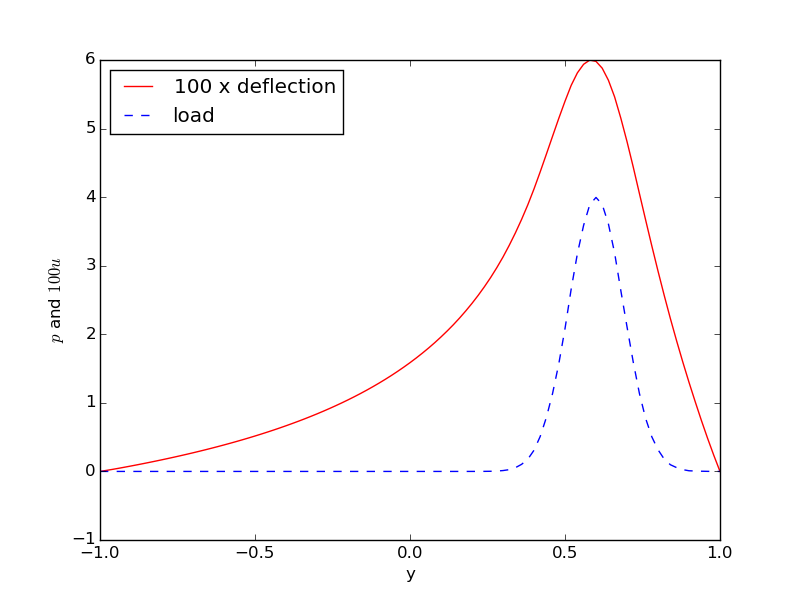## Visualizing the solution in ParaView

ParaView is a powerful tool for visualizing scalar and vector fields, including those computed by FEniCS.

Our program writes the fields $$w$$ and $$p$$ to file as finite element functions. We choose the names of these files to be membrane_deflection.pvd for $$w$$ and membrane_load.pvd for $$p$$. These files are in VTK format and their data can be visualized in ParaView. We now give a detailed account for how to visualize the fields $$w$$ and $$p$$ in ParaView.

1. Start the ParaView application.
2. Open a file with File - Open.... You will see a list of .pvd and .vtu files. More specifically you will see membrane_deflection.pvd. Choose this file.
3. Click on Apply to the left (Properties pane) in the GUI, and ParaView will visualize the contents of the file, here as a color image.
4. To get rid of the axis in the lower left corner of the plot area and axis cross in the middle of the circle, find the Show Orientation Axis and Show Center buttons to the right in the second row of buttons at the top of the GUI. Click on these buttons to toggle axis information on/off.
5. If you want a color bar to explain the mapping between $$w$$ values and colors, go to the Color Map Editor in the right of the GUI and use the Show/hide color legend button. Alternatively, find Coloring in the lower left part of the GUI, and toggle the Show button.
6. The color map, by default going from blue (low values) to red (high values), can easily be changed. Find the Coloring menu in the left part of the GUI, click Edit, then in the Color Map Editor double click at the left end of the color spectrum and choose another color, say yellow, then double click at the right and of the spectrum and choose pink, scroll down to the bottom of the dialog and click Update. The color map now goes from yellow to pink.
7. To save the plot to file, click on File - Export Scene..., fill in a filename, and save. See Figure 5 (middle).
8. To change the background color of plots, choose Edit - Settings..., Color tab, click on Background Color, and choose it to be, e.g., white. Then choose Foreground Color to be something different.
9. To plot the mesh with colors reflecting the size of $$w$$, find the Representation drop down menu in the left part of the GUI, and replace Surface by Wireframe.
10. To overlay a surface plot with a wireframe plot, load $$w$$ and plot as surface, then load $$w$$ again and plot as wireframe. Make sure both icons in the Pipeline Browser in the left part of the GUI are on for the membrane_deflection.pvd files you want to display. See Figure 5 (left).
11. Redo the surface plot. Then we can add some contour lines. Press the semi-sphere icon in the third row of buttons at the top of the GUI (the so-called filters). A set of contour values can now be specified at in a dialog box in the left part of the GUI. Remove the default contour (0.578808) and add 0.01, 0.02, 0.03, 0.04, 0.05. Click Apply and see an overlay of white contour lines. In the Pipeline Browser you can click on the icons to turn a filter on or off.
12. Divide the plot window into two, say horizontally, using the top right small icon. Choose the 3D View button. Open a new file and load membrane_load.pvd. Click on Apply to see a plot of the load.
13. To plot a 2D scalar field as a surface, load the field, click Apply to plot it, then select from the Filters pulldown menu the filter Warp By Scalar, click Apply, then toggle the 2D button to 3D in the Layout #1 window (upper row of buttons in that window). Now you can rotate the figure. The height of the surface is very low, so go to the Properties (Warp By Scalar1) window to the left in the GUI and give a Scale Factor of 20 and re-click Apply to lift the surface by a factor of 20. Figure 5 (right) shows the result.

Figure 5: Default visualizations in ParaView: deflection (left, middle) and pressure load (right).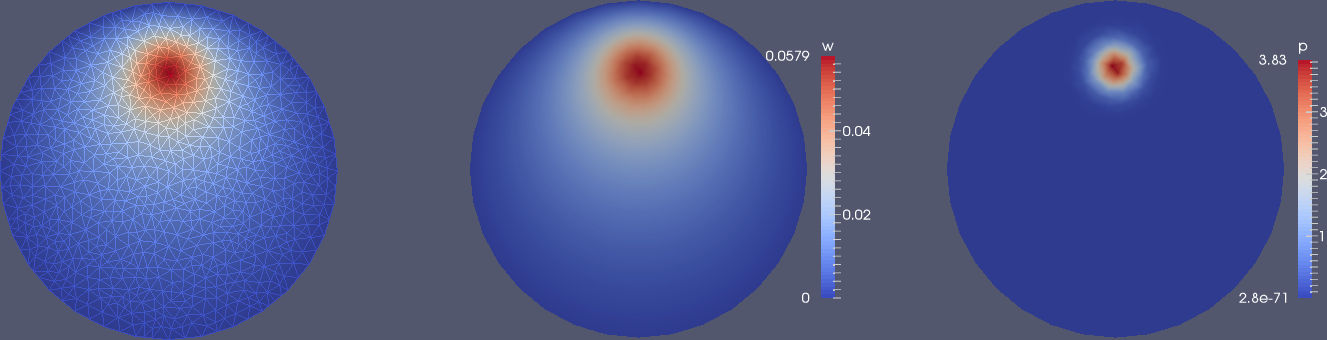Figure 6: Use of Warp By Scalar filter to create lifted surfaces (with different vertical scales!) in ParaView: load (left) and deflection (right).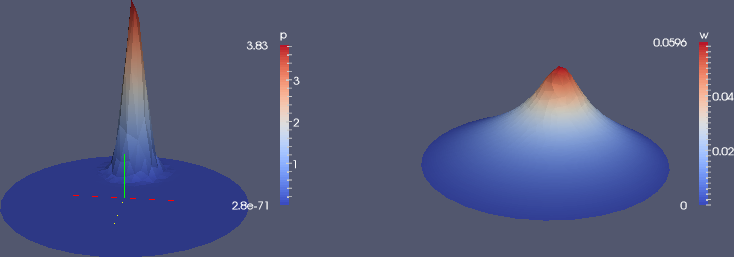A particularly useful feature of ParaView is that you can record GUI clicks (Tools - Start/Stop Trace) and get them translated to Python code. This allows you automate the visualization process. You can also make curve plots along lines through the domain, etc.

For more information, we refer to The ParaView Guide  (free PDF available) and to the ParaView tutorial as well as an instruction video.

## Using the built-in visualization tool

This section explains some useful visualization features of the built-in visualization tool in FEniCS. The plot command applies the VTK package to visualize finite element functions in a very quick and simple way. The command is ideal for debugging, teaching, and initial scientific investigations. The visualization can be interactive, or you can steer and automate it through program statements. More advanced and professional visualizations are usually better created with advanced tools like Mayavi, ParaView, or VisIt.

The plot function can take additional arguments, such as a title of the plot, or a specification of a wireframe plot (elevated mesh) instead of a colored surface plot:

plot(mesh, title='Finite element mesh')
plot(w, wireframe=True, title='Solution')


Axes can be turned on by the axes=True argument, while interactive=True makes the program hang at the plot command - you have to type q in the plot window to terminate the plot and continue execution.

The left mouse button is used to rotate the surface, while the right button can zoom the image in and out. Point the mouse to the Help text down in the lower left corner to get a list of all the keyboard commands that are available.

The plots created by pressing p or P are stored in filenames having the form dolfin_plot_X.png or dolfin_plot_X.pdf, where X is an integer that is increased by one from the last plot that was made. The file stem dolfin_plot_ can be set to something more suitable through the hardcopy_prefix keyword argument to the plot function, for instance, plot(f, hardcopy_prefix='pressure').

The ranges of the color scale can be set by the range_min and range_max keyword arguments to plot. The values must be float objects. These arguments are important to keep fixed for animations in time-dependent problems.

Figure 7: Plot of the deflection of a membrane using the built-in visualization tool.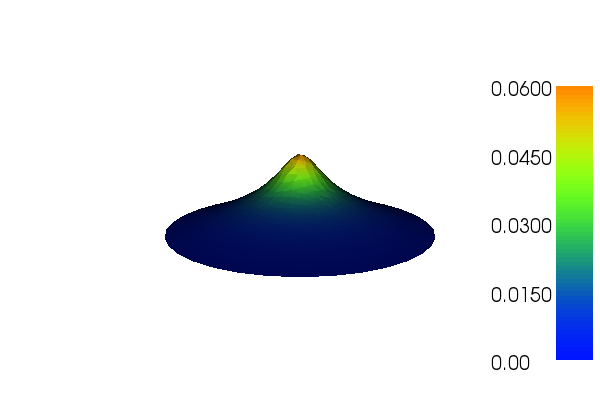Built-in plotting on Mac OS X and in Docker. The built-in plotting in FEniCS may not work as expected when either running on Mac OS X or when running inside a FEniCS Docker container. FEniCS supports plotting using the plot() command on Mac OS X. However, the keyboard shortcuts h, p, P and so on may fail to work. When running inside a Docker container, plotting is not supported since Docker does not interact with your windowing system. For Docker users who need plotting, it is recommended to either work within a Jupyter/FEniCS notebook (command fenicsproject notebook) or rely on Paraview or other external tools for visualization.

## Exercise 1: Visualize a solution in a cube

(hpl 2: The solution refers to a solver function. This is not introduced before later, so present both a flat program and a solver function (as a teaser).)

Solve the problem $$-\nabla^2 u = f$$ on the unit cube $$[0,1]\times[0,1]\times [0,1]$$ with $$u_0 = 1 + x^2 + 2y^2 - 4z^2$$ on the boundary. Visualize the solution. Explore both the built-in visualization tool and ParaView.

Solution.

As hinted by the filename in this exercise, a good starting point is the solver function in the program ft03_poisson_function.py, which solves the corresponding 2D problem. Only two lines in the body of solver needs to be changed (!): mesh = .... Replace this line with

mesh = UnitCubeMesh(Nx, Ny, Nz)


and add Nz as argument to solver. We implement the new $$u_0$$ function in application_test and realize that the proper $$f(x,y,z)$$ function in this new case is 2.

u0 = Expression('1 + x*x + 2*x*x - 4*x*x')
f = Constant(2.0)
u = solver(f, u0, 6, 4, 3, 1)


The numerical solution is without approximation errors so we can reuse the unit test from 2D, but it needs an extra Nz parameter.

The variation in $$u$$ is only quadratic so a coarse mesh is okay for visualization. Below is plot from the ParaView (left) and the built-in visualization tool (right). The usage is as in 2D, but now one can use the mouse to rotate the 3D cube.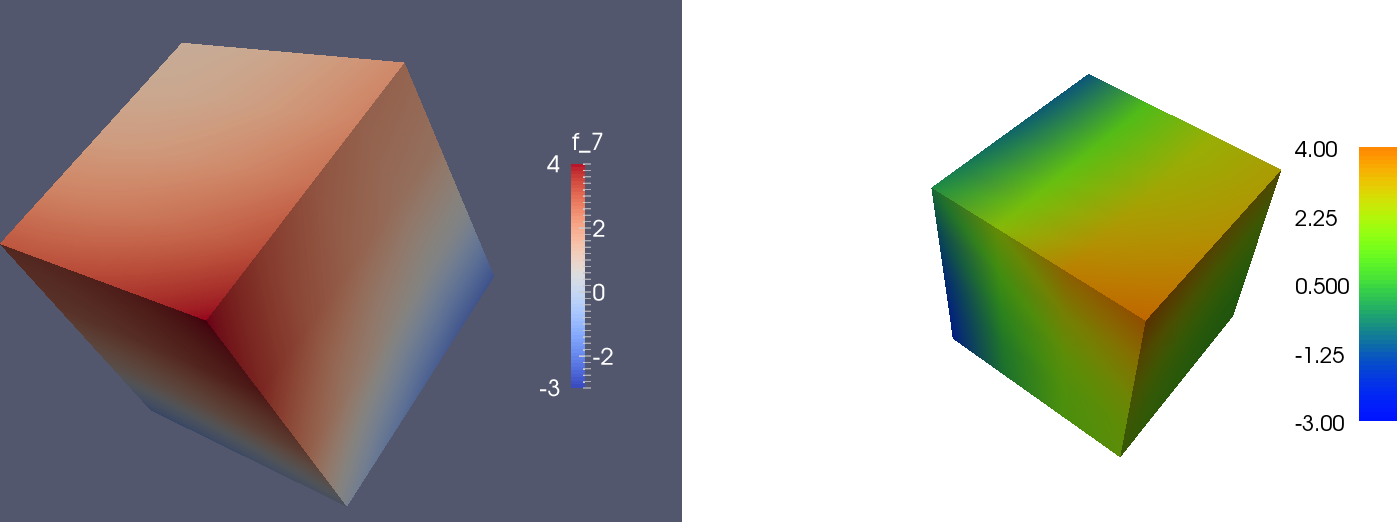We can in ParaView add a contour filter and define contour surfaces for $$u=-2,1,0,1,2,3$$, then add a slice filter to get a slice with colors: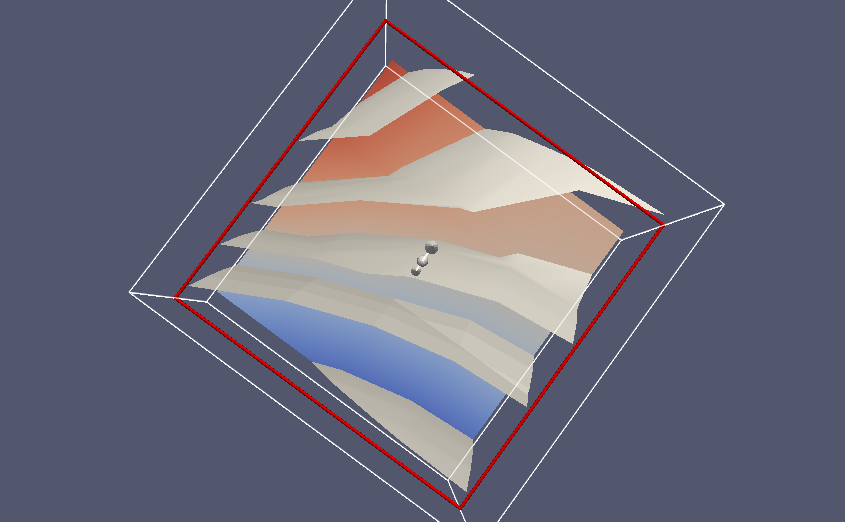Filename: poissin_3d_func.